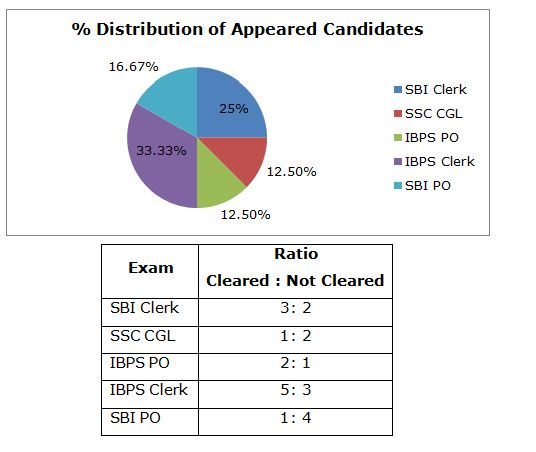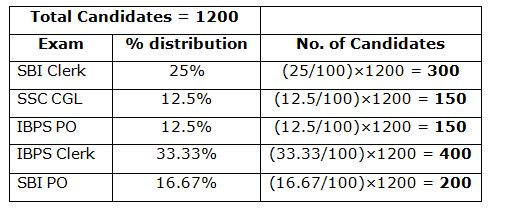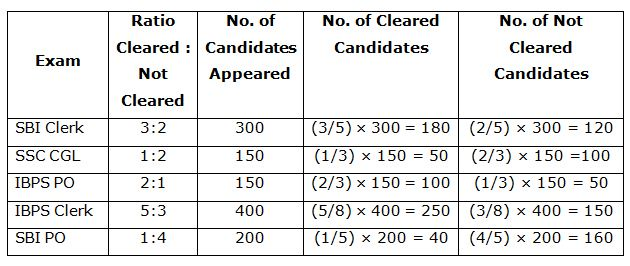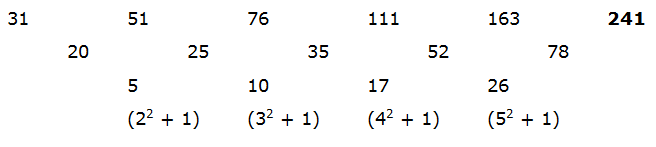# SBI Clerk Prelims 2021 Quantitative Aptitude Questions (Day-07)

Dear Aspirants, Our IBPS Guide team is providing new series of Quantitative Aptitude Questions for SBI Clerk Prelims 2020 so the aspirants can practice it on a daily basis. These questions are framed by our skilled experts after understanding your needs thoroughly. Aspirants can practice these new series questions daily to familiarize with the exact exam pattern and make your preparation effective.

Start Quiz

Data Interpretation

Directions (1-5): Study the following information carefully and answer the questions given below.

The total number of candidates appeared from ABC academy is 1200.  The data regarding the distribution in percentage of total number of students appeared for various banking competitive exams are given in the following Pie chart.

Also the table shows the ratio between numbers of candidates cleared and not cleared the various exams.1) What is the difference between the number of candidates cleared SBI PO & SBI clerk together and number of candidates cleared IBPS PO & IBPS clerk together?

A.150

B.80

C.130

D.50

E.None of these

2) What is the ratio of the number of candidates not cleared SSC CGL to who cleared SBI PO?

A.1: 20

B.5: 8

C.5: 2

D.5: 4

E.None of these

3) The total number of candidates cleared all exams is what percent of total number of candidates not cleared?

A.106.89 %

B.93.54 %

C.51.66 %

D.48.33 %

E.None of these

4) What is the central angle formed by number of candidates appeared SBI Clerk and SSC CGL together?

A.108°

B.135°

C.144°

D.54°

E.None of these

5) What is the ratio of the total number of candidates cleared and those who not cleared all types of exams?

A.31/60

B.29/31

C.31/29

D.29/60

E.None of these

Missing number series

Directions (6-10): Find out the missing number in the following number series.

6) 1, 5, 21, ?, 121

A.57

B.70

C.85

D.101

E.104

7) 31, 51, 76, 111, 163, ?

A.249

B.241

C.271

D.281

E.None of these

8) 3390, 2758, ?, 1740, 1342

A.1018

B.2208

C.2210

D.1902

E.None of these

9)
1, 2, 6, 21,?

A.108

B.98

C.88

D.89

E.None of these

10) 1, 1.5, 4.5, 20.25, ?

A.82

B.121.5

C.101.25

D.142.75

E.None of these

Directions (1-5) :

The number of candidates appeared out of 1200 as per Pie chart:The number of candidates Cleared & not cleared as per table chart:Total candidates cleared SBI PO + SBI Clerk = 180 + 40 = 220

Total candidates cleared IBPS PO + IBPS Clerk = 100 + 250 = 350

Therefore, Difference = 350 – 220 = 130

Total candidates not cleared SSC CGL = 100

Total candidates cleared SBI PO = 40

Therefore, Ratio = 100: 40 = 5: 2

Total candidates cleared = 180 + 50 + 100 + 250 + 40 = 620

Total candidates not cleared = 1200 – 620 = 580

Therefore, % = (620/580) × 100 = 106.89%

Total Pie chart angle = 360°

% of SBI Clerk + SSC CGL in Pie chart = 25% + 12.5% = 37.5%

Therefore, req. central angle = 37.5% of 360° = (37.5/100) × 360° = 135°

Total candidates cleared = 180+50+100+250+40= 620

Total candidates not cleared = 1200 – 620 = 580

Therefore, Ratio = 620/580 = 31/29

1 + 4(22) = 5

5 + 16(42) = 21

21 + 36(62) = 57

57 + 64(82) = 1213390 = 153 + 15

2758 = 143 + 14

2210 = 133 + 13

1740 = 123 + 12

(1 × 1) + 1 = 2

(2 × 2) + 2 = 6

(6 × 3) + 3 = 21

(21 × 4) + 4 = 88

1 × 1.5 = 1.5

1.5 × 3 = 4.5

4.5 × 4.5 = 20.25

20.25 × 6 = 121.5

 Check Here to View SBI Clerk Prelims 2021 Quantitative Aptitude Questions Day – 06 Day – 05 Day – 04
5 1 vote
Rating